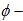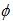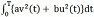##### Constancy of Holomorphic Sectional Curvature for Indefinite S- Manifolds

Jae Won Lee

Journal of Advances in Mathematics and Computer Science, Page 121-128
DOI: 10.9734/BJMCS/2011/270

Kumar et al. (2009) provided an algebraic characterization for an indefinite Sasakian manifold to

reduce to a space of constantholomorphic sectional curvature. In this present paper, we

generalize the characterization for indfinite S -manifolds, which is a space of constantholomorphic sectional curvature if and only if R (X ,X) X is proportional toX.

##### The Price of Asset-liability Control under Tail Conditional Expectation with No Transaction Cost

Bright O. Osu

Journal of Advances in Mathematics and Computer Science, Page 129-140
DOI: 10.9734/BJMCS/2011/355

Asset-liability management is a means of managing the risk that can arise from the changes in the relationship between assets and liabilities. Value-at-risk (VaR) and tail conditional expectation (TCE) have also emerged in recent years as standard tools for measuring and controlling the risk of trading portfolios. In some dynamical settings however, the limits of TCE can be transformed into the limits of VaR and conversely even though TCE is more preferable to VaR  since it is coherent and VaR is not. In this paper we obtain the optimal price of an institution’s assets- liabilities under the TCE with no transaction cost.

##### Modification of Einstein’s Gravitational Field Equation and Cracking of Foundational Difficulties in Physics

Jian Liang Yang

Journal of Advances in Mathematics and Computer Science, Page 141-164
DOI: 10.9734/BJMCS/2011/294

In the framework of gravitational theory of general relativity, this article has systematically and radically solved the problem of galaxy formation and some significant cosmological puzzles. A flaw with Einstein’s equation of gravitational field is firstly corrected and the foundations of general relativity are perfected and developed, and space-time is proved to be infinite, expansion and contraction of universe are proved to be in circles, the singular point of big bang is naturally eliminated, celestial bodies and galaxies are proved growing up with cosmic expansion, for example Earth’s mass and radius at present increase by 1.2 trillion tons and 0.45mm, respectively in a year, in response to which geostationary satellites rise by 2.7mm.

##### Comparative Analysis of Extended Conjugate Method (ECGM) and Euler-Lagrange (E-L) Algorithms for Reaction Diffusion Problem

J. O. Omolehin, K. Rauf, O. Abikoye, O. Enikuomehin, S. O. Sogunro

Journal of Advances in Mathematics and Computer Science, Page 165-180
DOI: 10.9734/BJMCS/2011/159

Reaction Diffusion Control problem is a class of optimization problem in the form

MinimizeSubject toIt has been proved that a unique solution exists. In this work, the solution provided by ECGM

and Euler-Lagrange algorithm, are admissible and favourably comparable.